Browse by Topic

# Building Uniform Polyhedra for Version 12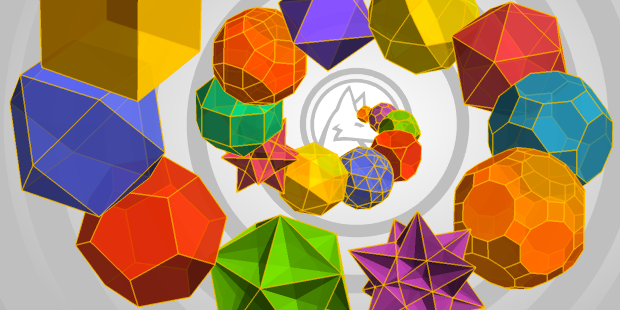Since I started working at Wolfram, I’ve been a part of several different projects. For Version 12, my main focus was replicating models of the uniform polyhedra with the Wolfram Language to ensure that the data fulfilled certain criteria to make our models precise, including exact coordinates, consistent face orientation and a closed region in order to create a proper mesh model of each solid.

Working with visual models of polyhedra is one thing, but analyzing them mathematically proved to be much more challenging. Starting with reference models of the polyhedra, I found that the Wolfram Language made mathematical analysis of uniform polyhedra particularly efficient and easy.

But first, what really are polyhedra, and why should we care? With Version 12, we can explore what polyhedra are and how they’ve earned their continued place in our imaginations.

## What Are Polyhedra?

Polyhedra are 3D solids composed of flat polygonal faces. Adjacent faces meet at edges, and edges meet at vertices. The fascination with polyhedra goes beyond just mathematicians. The ancient Greeks proved that there were five regular polyhedra, or the five Platonic solids: Tetrahedron, Cube, Octahedron, Dodecahedron and Icosahedron—all newly introduced in Version 12: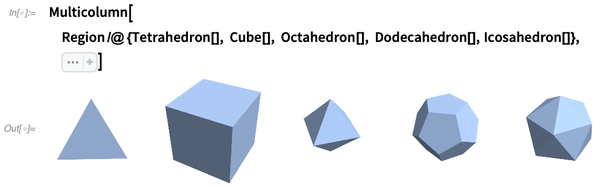✕ ```Multicolumn[ Region /@ {Tetrahedron[], Cube[], Octahedron[], Dodecahedron[], Icosahedron[]}, Sequence[5, Spacings -> 1]]```

Sixteenth-century astronomer Johannes Kepler even based a solar system on polyhedra by trying to find a relationship between the ratios of the orbits of the six planets known at his time and the ratios of the Platonic solids.

This model shows the Platonic solids embedded inside spheres, where each of the solids is touching two planetary spheres. Kepler believed this explained the distance between the planets and why there were exactly six planets: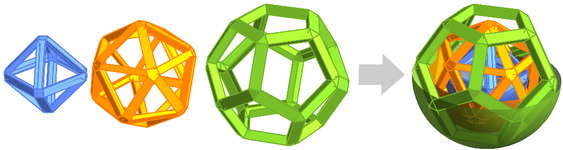Still, it wasn’t until Leonhard Euler that an important formula regarding polyhedra was discovered, stating that the number of vertices minus the number of edges plus the number of faces is equal to 2 in a polyhedron:

V – E + F = 2

The left-hand side of this equality is known as the Euler–Poincaré characteristic, which we can test using EulerCharacteristic in Version 12: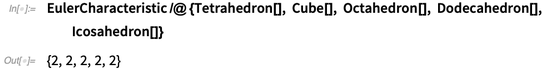✕ ```EulerCharacteristic /@ {Tetrahedron[], Cube[], Octahedron[], Dodecahedron[], Icosahedron[]}```

At the time, the theory of polyhedra mainly focused on properties such as measuring angles, finding the areas of faces and finding the lengths of sides. Euler instead started to classify these solids by counting their different features. In correspondence with Christian Goldbach, Euler talked about what he considered to be the important parts of the polyhedron: the faces, vertices and edges. In this way, not only did Euler derive the famous Euler–Poincaré characteristic, but he also paved the way for the origin of topology; instead of addressing distance, as traditional geometry does, he used other properties to describe a surface, as topology does.

## What Really Is a Polyhedron?

While modeling a geometric shape may seem like a straightforward task, there are in fact some unique complications that must be considered. There have always been conflicting views on what is to be called a polyhedron. As mentioned before, a polyhedron at its most basic definition is a solid consisting of vertices, edges and faces. However, there’s still no universal agreement about what a polyhedron is. Some say that polyhedra include both convex and nonconvex cases, while others will only define convex cases as polyhedra.

There are many other aspects of polyhedra that are debated among people who study them. What happens when there are intersecting polygons inside a polyhedron? Should what happens on the inside of the models be taken into account? What about uniform polyhedra that use pentagrams and other polygons—for which there are also debates? Should those models be depicted with voids? Opposing views such as these make it even more difficult to create models of polyhedra.

## Uniquely Uniform

There are many different polyhedra, but this post will focus on 75 special polyhedra, more commonly referred to as the uniform polyhedra. The uniform polyhedra are vertex-transitive and only have two faces per edge; more importantly, all the polygons composing these polyhedra are regular. With Version 12, we can now use EntityList and UniformPolyhedron to provide us with information about the uniform polyhedra: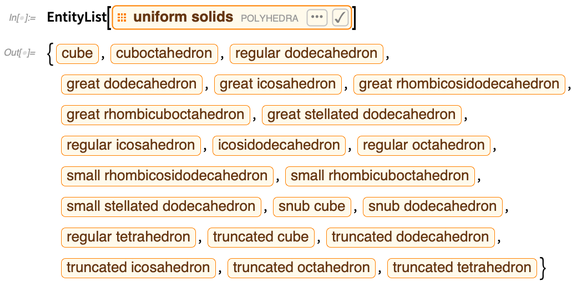✕ ```EntityList[\!\(\*NamespaceBox["LinguisticAssistant", DynamicModuleBox[{Typeset`query\$\$ = "uniform polyhedra", Typeset`boxes\$\$ = TemplateBox[{"\"uniform solids\"", RowBox[{"EntityClass", "[", RowBox[{"\"Polyhedron\"", ",", "\"Uniform\""}], "]"}], "\"EntityClass[\\\"Polyhedron\\\", \\\"Uniform\\\"]\"", "\"polyhedra\""}, "EntityClass"], Typeset`allassumptions\$\$ = {{"type" -> "Clash", "word" -> "uniform polyhedra", "template" -> "Assuming \"\${word}\" is \${desc1}. Use as \${desc2} instead", "count" -> "2", "Values" -> {{"name" -> "PolyhedronClass", "desc" -> "a class of polyhedra", "input" -> "*C.uniform+polyhedra-_*PolyhedronClass-"}, {"name" -> "MathWorld", "desc" -> " referring to a mathematical definition", "input" -> "*C.uniform+polyhedra-_*MathWorld-"}}}}, Typeset`assumptions\$\$ = {}, Typeset`open\$\$ = {1, 2}, Typeset`querystate\$\$ = {"Online" -> True, "Allowed" -> True, "mparse.jsp" -> 0.29795`5.925688383256781, "Messages" -> {}}}, DynamicBox[ ToBoxes[AlphaIntegration`LinguisticAssistantBoxes["", 4, Automatic, Dynamic[Typeset`query\$\$], Dynamic[Typeset`boxes\$\$], Dynamic[Typeset`allassumptions\$\$], Dynamic[Typeset`assumptions\$\$], Dynamic[Typeset`open\$\$], Dynamic[Typeset`querystate\$\$]], StandardForm], ImageSizeCache -> {224., {7., 15.}}, TrackedSymbols :> {Typeset`query\$\$, Typeset`boxes\$\$, Typeset`allassumptions\$\$, Typeset`assumptions\$\$, Typeset`open\$\$, Typeset`querystate\$\$}], DynamicModuleValues :> {}, UndoTrackedVariables :> {Typeset`open\$\$}], BaseStyle -> {"Deploy"}, DeleteWithContents -> True, Editable -> False, SelectWithContents -> True]\)]```

## Extracting Information from Models

Models generally found for polyhedra look like these: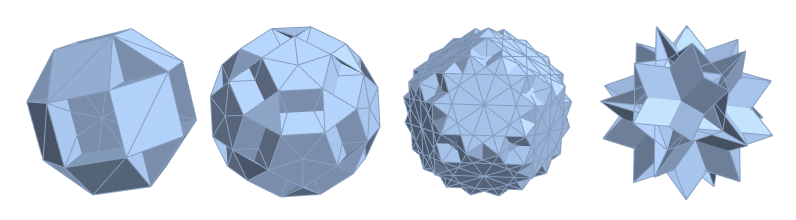These are graphical representations of the uniform polyhedra. Prior to Version 12, PolyhedronData had information for some of these polyhedra. In Version 12, we can now represent the first model as a Polyhedron with 72 faces and 30 vertices: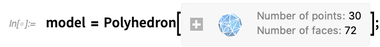✕ `model = CloudGet["https://wolfr.am/FjduaZOs"]`

By using FaceForm, we can see the orientation of the faces is flipped in some areas: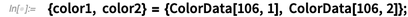✕ `{color1, color2} = {ColorData[106, 1], ColorData[106, 2]};`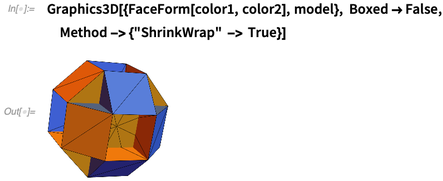✕ ```Graphics3D[{FaceForm[color1, color2], model}, Boxed -> False, Method -> {"ShrinkWrap" -> True}]```

The model has crossing polygons and breaks the “right-hand rule” of polygons (shown here), meaning it has incorrect face orientation: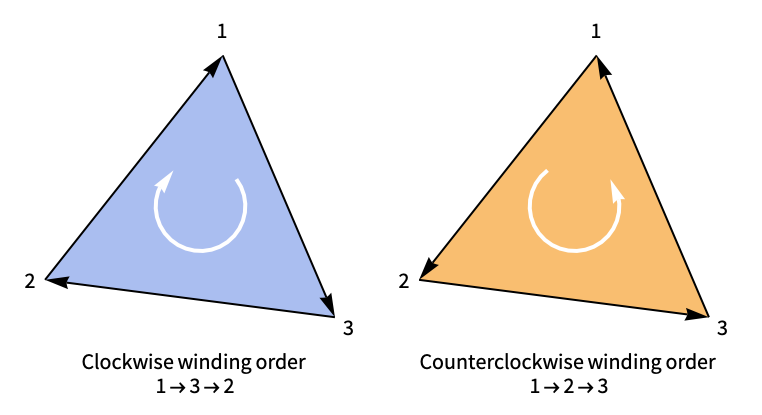In order to replicate the polyhedra for this project, changes needed to be made to these models to resolve these issues. My first approach was simply to use the standard models and obtain vertices from them to recreate the polyhedra, and then change the orientation of the faces to follow the right-hand rule. The next step would be to scale the models to unit size and gather the exact coordinates. Unfortunately, obtaining exact coordinates from these models was no easy feat; after several failed attempts at obtaining the exact coordinates using these models, it was time to go back and learn more about polyhedra.

## Creating the Solids

Some polyhedra share vertex and edge arrangements. Thus, I could recreate the polyhedra that share properties by gathering the data of the uniform polyhedra available in PolyhedronData. From this, I was able to compile a list of the uniform polyhedra and their “siblings,” and thus was able to “modify” the faces and create the polyhedra.

Let’s try it out by creating the small rhombihexahedron. To do so, take the vertex and shared face data (in this case, 12 square faces) from the small rhombicuboctahedron from PolyhedronData and use FaceForm to make sure all the faces follow the right-hand rule: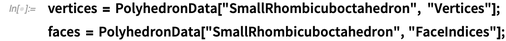✕ ```vertices = PolyhedronData["SmallRhombicuboctahedron", "Vertices"]; faces = PolyhedronData["SmallRhombicuboctahedron", "FaceIndices"];```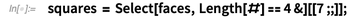✕ `squares = Select[faces, Length[#] == 4 &][[7 ;;]];`

I end up with the following “shell” of a polyhedron: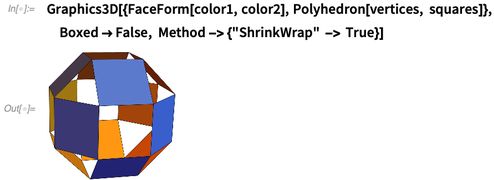✕ ```Graphics3D[{FaceForm[color1, color2], Polyhedron[vertices, squares]}, Boxed -> False, Method -> {"ShrinkWrap" -> True}]```

Compared to the first model, we can see that the octagon faces are missing in the shell. Those faces can be defined by visually comparing the first model to the shell: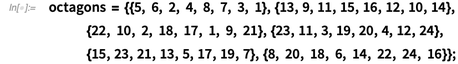✕ `octagons = {{5, 6, 2, 4, 8, 7, 3, 1}, {13, 9, 11, 15, 16, 12, 10, 14}, {22, 10, 2, 18, 17, 1, 9, 21}, {23, 11, 3, 19, 20, 4, 12, 24}, {15, 23, 21, 13, 5, 17, 19, 7}, {8, 20, 18, 6, 14, 22, 24, 16}};`

Now we add the new octagon faces to our shell: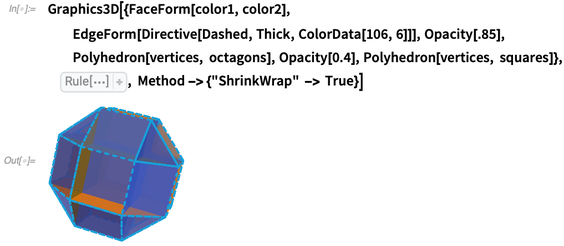✕ ```Graphics3D[{FaceForm[color1, color2], EdgeForm[Directive[Dashed, Thick, ColorData[106, 6]]], Opacity[.85], Polyhedron[vertices, octagons], Opacity[0.4], Polyhedron[vertices, squares]}, Boxed -> False, Method -> {"ShrinkWrap" -> True}] ```

The new small rhombihexahedron is created!

However, this model also has flipped faces. When creating the polyhedra, it is not often taken into account that some faces can be seen from both sides, resulting in flipped faces. One way of fixing this issue is to split the polygons and follow the right-hand rule. In this case, octagons now become triangles and rectangles: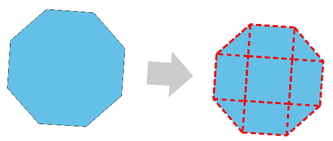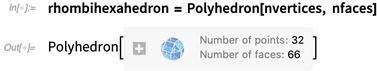✕ `rhombihexahedron = Polyhedron[nvertices, nfaces]`

The small rhombihexahedron is complete! Using FaceForm, we can check that the model has the correct face orientation: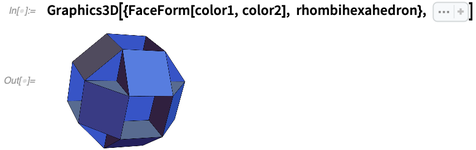✕ ```Graphics3D[{FaceForm[color1, color2], rhombihexahedron}, Sequence[ Boxed -> False, Method -> {"ShrinkWrap" -> True}]] ```

The issues with face orientation are no longer in the model, and thus this process can be repeated for a majority of the uniform polyhedra.

More complex models need a different approach, and for those, we’ll use a binary space partitioning (BSP) tree. With the exact coordinates and the faces, we can recreate the polyhedra, but it’s not always so clear how to ensure that all the faces are in the correct orientation. With polyhedra such as the small rhombihexahedron, it is easy to visualize where the faces should be split and maintain consistency across the polyhedron. With others, such as the great icosihemidodecahedron, it is not as simple to decide where those should be. Thus, using a BSP tree allows us to see where the different faces of the mesh could be split.

Here we have the model of the great icosihemidodecahedron without any alterations, using the exact coordinates from its convex hull, the icosahedron and its shared-edge arrangements with the great icosidodecahedron (triangular faces) and with the great dodecahemidodecahedron (decagram faces):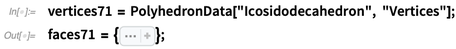✕ ```vertices71 = PolyhedronData["Icosidodecahedron", "Vertices"]; faces71 = { Sequence[{20, 15, 16, 21, 1, 23, 19, 18, 22, 2}, {12, 5, 6, 13, 1, 8, 28, 27, 7, 2}, {10, 7, 20, 4, 14, 25, 13, 23, 29, 11}, {21, 3, 14, 26, 12, 22, 30, 11, 9, 8}, {6, 17, 18, 30, 10, 27, 24, 16, 3, 25}, {9, 29, 19, 17, 5, 26, 4, 15, 24, 28}, {13, 23, 1}, {22, 12, 2}, {20, 7, 2}, {21, 16, 3}, {14, 25, 3}, {26, 14, 4}, {15, 20, 4}, {17, 6, 5}, {26, 12, 5}, {13, 25, 6}, {27, 10, 7}, {21, 1, 8}, {9, 28, 8}, {29, 11, 9}, {11, 30, 10}, {16, 24, 15}, {18, 19, 17}, {22, 30, 18}, {29, 23, 19}, {28, 27, 24}]};```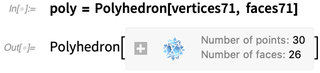✕ `poly = Polyhedron[vertices71, faces71]`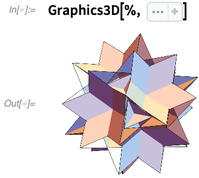✕ ```Graphics3D[%, Sequence[ Boxed -> False, Method -> {"ShrinkWrap" -> True}]]```

Using FaceForm, we can see that the model also has flipped faces: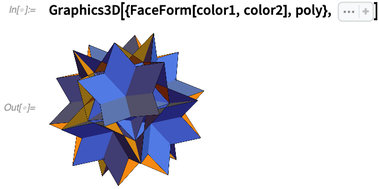✕ ```Graphics3D[{FaceForm[color1, color2], poly}, Sequence[ Boxed -> False, Method -> {"ShrinkWrap" -> True}]]```

These problems can be “fixed” in a similar way as the small rhombihexahedron. However, it is difficult to see where faces should be split. This is where the BSP tree comes in, because it will allow a closer look and a cleaner distinction as to where the splitting of the polygon faces should be, as well as provide the coordinates needed to create the new splits.

Using the BSP tree, this mesh is converted to a subdivided mesh region, which can then be used to extract the necessary information.

Once the mesh is partitioned, use Graphics3D to see the current face orientation and gather where the splits should be made: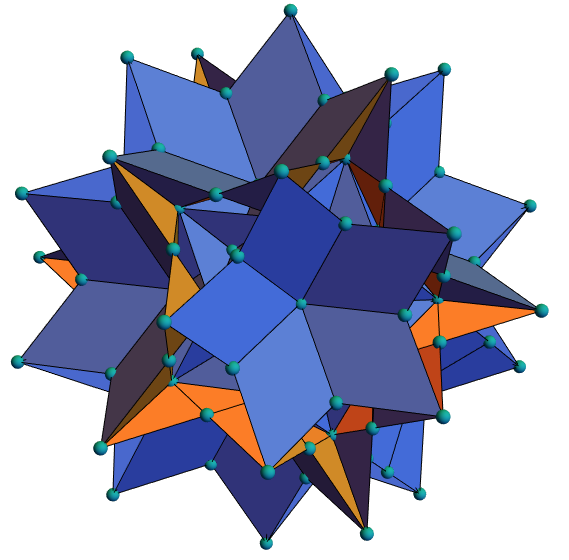Once it is determined where the faces can be split, the coordinates can be extracted from the resulting mesh of the BSP tree.

## Cutting Corners?

While we have the exact coordinates for all the uniform polyhedra, the intersecting faces in some of the polyhedra make it difficult to determine where polygons should be split, especially in the nonconvex cases.

The snub dodecadodecahedron, the great retrosnub icosidodecahedron and the great dirhombicosidodecahedron are good examples of difficult polyhedra to split. Both visually and computationally, it is difficult to find where the faces need to be split in order to produce a precise copy of the original model with exact coordinates and the proper face orientation. This is especially hard with the vast amount of overlapping faces, holes from star polygons and crevices that are difficult to distinguish, even after BSP tree analysis: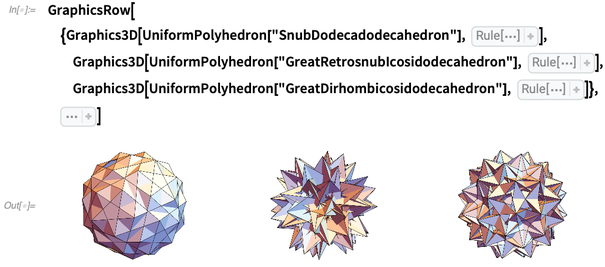✕ ```GraphicsRow[{Graphics3D[UniformPolyhedron["SnubDodecadodecahedron"], Boxed -> False], Graphics3D[UniformPolyhedron["GreatRetrosnubIcosidodecahedron"], Boxed -> False], Graphics3D[UniformPolyhedron["GreatDirhombicosidodecahedron"], Boxed -> False]}, Sequence[0, ImageSize -> Full]]```

This was when I decided to go back to the basics and make these polyhedra by hand. I wanted to avoid printing out a net of the polyhedra that I was making. If I used nets, I would not necessarily run into the issues that I was coming across computationally. To do this, I cut out the polygons necessary (and then some) to make the polyhedra. For the small rhombihexahedron, I used a two-inch scale to make the necessary squares and octagons.

Right away, I ran into one of the issues that I had computationally: intersecting polygons. This can be resolved by making slits in the polygons so that they are able to come together. However, I quickly realized that the octagon faces would need more than one split, and after some polygon splitting, I was able to build the octagon framework of the small rhombihexahedron. Applying the square faces was the last thing to do to finish the small rhombihexahedron: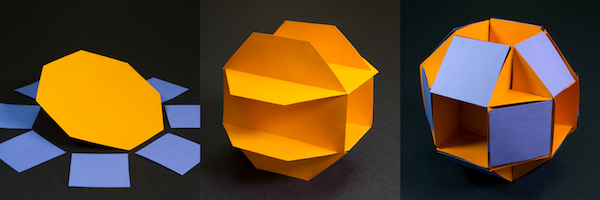Using the newly split small rhombihexahedron, I was also able to 3D print the model for a comparison with the paper model: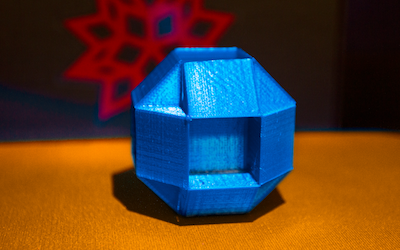From my experience building these by hand, it was necessary to split polygons to bring the final polyhedron together.

After getting comfortable with some of the polyhedra, I could now try my hand at the ones that defeated me computationally, like the great rhombicosidodecahedron. I’m discovering new perspectives as I make the polyhedra, though there are still some challenges to tackle—even the paper models for those polyhedra are difficult to make, and the splitting of polygons is not always clear. We’re continually working on getting all the uniform polyhedra into the Wolfram Language—so keep an eye out for the great inverted snub icosidodecahedron!

 Get full access to the latest Wolfram Language functionality for geometry—including polygons and polyhedra—with Mathematica 12. View plans